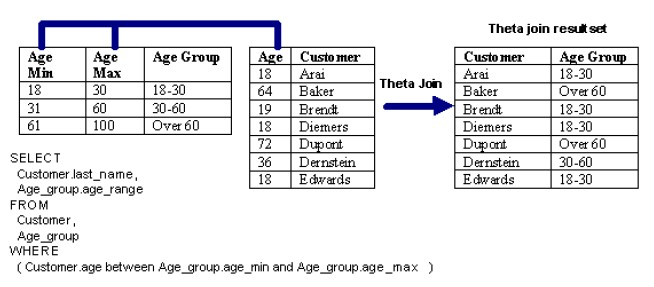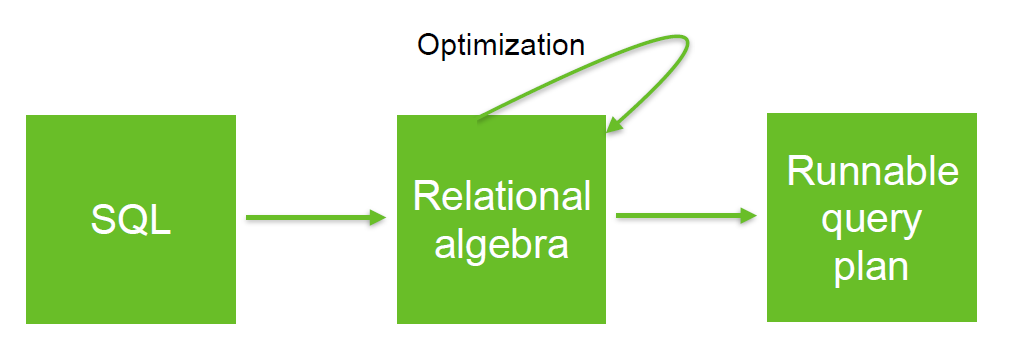# Relational Algebra - Theta-join

A theta-join is a difficult/complex join where the condition is not a equality.

Example:

• Band join
• or range join.

A theta is a join that links tables based on a relationship other than the equality between two columns.

A theta join could use any other operator than the equal operator.

A theta may not have any join key in the sql but you still have a join physically (ie when running the sql).

## Notation

Algebraic rule

Physically, the join of R1 and R2 with the condition theta is the same than the selection of the cross product of R1 and R2 with the condition theta.

## Example

### Find all the hospitals within 5 miles of a school

``````select distinct h.name
from hospitals h, schools s
where
distance(h.location,s.location) < 5```
```

where: Distance

### Find all user clicks made within 5 seconds of page load

``````select *
where
```

Discover MoreBOBJ - Theta joins

in BOBJDistance

is a numerical description of how far apart objects are. Same as: In most cases, “distance from A to B” is interchangeable with “distance between B and A”. In physics or everyday...Redis

is an open source (BSD licensed), in-memory key-value data store used as: database, cache and message broker. Hash, (if you have objects representing users in a web application,...Relational Operator - Equi-joins

An Equi-join is a join where the condition (predicate) is an equality. theta join An equi-join links two relations (tables, ...) on common values in a column in relation 1 with a column in relation...Relational Operator - Join

join is a relational operator that pair tuples (row) of two relations (table, ...) according to a condition (predicate). where: c is a condition (ie predicate) See Type Symbol Unicode...